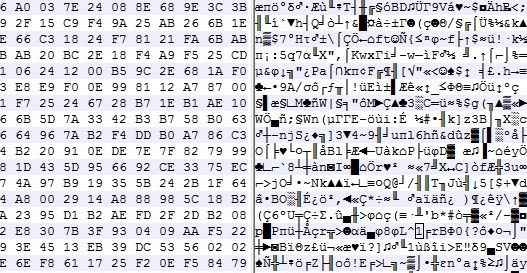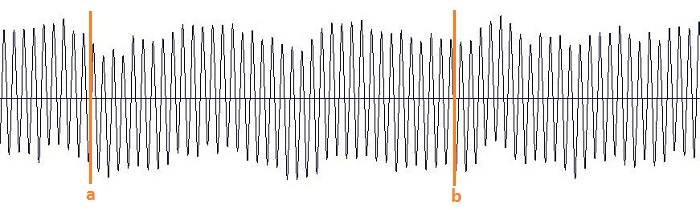email: here

#music
#photo
#opensource programming

Don't read #tech articles except you really want to.

Some of my projects:
BigPicture
SamplerBox
Ojourdui

23/10/2020

## An attempt to generate random data with audio (and your computer's built-in microphone)You probably know that generating some real random data is not so easy to do with a computer. How to design a good Random Number Generator (or a pseudo-random one) is a math topic that you can work years on ; it's also something very important for real-life applications such as security/cryptography, for example when you need to generate strong passwords.

Usually (and this is true in general in cryptography), designing your own algorithm is bad, because unless you're a professional in this subject and your algorithm has been approved by peers, you're guaranteed to have flaws in it, that could be exploited.

But here, for fun (don't use it for critical applications!), let's try to generate 100 MB of good random data.

1) Record 20 minutes of audio in 96khz 16bit mono with your computer's built-in microphone. Try to set the mic input level so that the average volume is neither 0 dB (saturation) nor -60 dB (too quiet). Something around -10 dB looks good. What kind of audio should you record? Nothing special, just the noise in your room is ok. You will get around 20*60*96000*2 ~ 220 MB of data. In these 220 MB, only the half will be really useful (because many values in the signal - an array of 16-bit integers - won't use the full 16-bit amplitude: many integers "encoding" the signal might be for example of absolute value < 1024, i.e. will provide only 10 bits)

2) Now let's shuffle these millions of bits of data with some Python code:

from scipy.io import wavfile
import numpy as np
import functools

#### GET A LIST OF ALL THE BITS
L = []  # list of bits
for i in range(len(x)):
bits = format(abs(x[i]), "b")  # get binary representation of the data
# don't use "016b" format because it would create a bias: small integers (those not using
# the full bit 16-bit amplitude) would have many leading 0s!
L += map(int, bits)[1:]        # discard the first bit, which is always 1!

print L.count(1)
print L.count(0)  # check if it's equidistributed in 0s and 1s

n = 2 ** int(np.log2(len(L)))
L = L[:n]  # crop the array of bits so that the length is a power of 2; well the only requirement is that len(L) is coprime with p (see below)

### RECREATE A NEW BINARY FILE WITH ALL THESE BITS (SHUFFLED)
# The trick is: don't use **consecutive bits**, as it would recreate something close to the input audio data.
# Let's take one bit every 96263 bits instead! Why 96263? Because it's a prime number, then we are guaranteed that
# 0 * 96263 mod n, 1 * 96263 mod n, 2 * 96263 mod n, ..., (n-1) * 96263 mod n will cover [0, 1, ..., n-1].  (**)
# This is true since 96263 is coprime with n. In math language: 96253 is a "generator" of (Z/nZ, +).

p = 96263  # The higher this prime number, the better the shuffling of the bits!
# If you have at least one minute of audio, you have at least 45 millions of useful bits already,
# so you could take p = 41716139 (just a random prime number I like around 40M)

M = set()
with open('random.raw', 'wb') as f:
for i in range(0, n, 8):
M.update(set([(k * p) % n for k in range(i, i+8)]))  # this is optional, here just to prove that our math claim (**) is true
c = [L[(k * p) % n] for k in range(i, i+8)]   # take 8 bits, in shuffled order
char = chr(functools.reduce(lambda a, b: a * 2 + b, c))  # create a char with it
f.write(char)

print M  == set(range(n))  # True, this shows that the assertion (**) before is true. Math rulez!

Done, your random.raw file is filled with random data!

Notes:

• The only issue I can see happen right now is if the ADC (analog-to-digital-converter) electronic component of your soundchip is highly biased (please drop me a message if you have such a device).

• This code here is unoptimized, it took 2 minutes for 1 minute of audio. There's surely a better way to work with arrays of bits in Python, comments/improvements are welcome!

• How to test the randomness quality of this file? This is a complicated task, and here are some references to do that. This is very far from being a rigorous way to do it, but it can be a first step (quote from the linked page): I've seen winzip used as a tool to measure the randomness of a file of values before (obviously, the smaller it can compress the file the less random it is). If you do it on the file generated here, you get exactly the same size (or even a bit more) after zip-compressing the file! Idem with rar, 7z (which usually yield a far better compression ratio, especially for audio data), the compression ratio is 1:1.

## How to find seamless loops in audio files (with a little bit of math and programming)

When making instrument sample sets (e.g. church organ sample sets used with Hauptwerk or GrandOrgue), we need to set looping points in WAV audio files:such that when playing the part [a, b] in loop, we don't hear any click or pop when the sample reaches the end of the loop.

Example 1: bad loop with audible clicks

Example 2: seamless loop with no click, that's what we are looking for! The loop has a ~ 2.670 second period, can you hear where are the looping points?

Finding looping points can be done manually but this is a very long and tedious task. A few programs exist to do this process automatically such as Extreme Sample Converter (it has an excellent auto-looping algorithm), LoopAuditioneer (open source), Zero-X Seamless Looper, SampleLooper, etc.

Here we'll look at a home-cooked algorithm that works well to detect looping points.

from scipy.io import wavfile
import numpy as np
import itertools

x0 = x if x.ndim == 1 else x[:, 0]     # let's keep only 1 channel for simplicity, but we could easily generalize this for 2 channels
x0 = np.asarray(x0, dtype=np.float32)

Let's say the audio file's sustain part (this is precisely where we're looking for a loop!) begins at t=2 sec and finishes at t=9 sec. We will now subdivide the time-interval [2 sec, 9 sec] into a 250 milliseconds grid: 2, 2.25, 2.5, 2.75, 3, 3.25, ..., 8.75, 9.

From this sequence, we now create "loop candidates" (a, b) of length at least 1 second, example: (2.5, 7.5), (3.25, 5.75), (6.0, 8.75), etc. Then, for each loop candidate, we'll improve the loop (this is the core of the algorithm, it will be discussed in the next paragraph) and compute a distance d. We finally keep the loop that has the minimal distance (among all loop candidates). Finished!

A = [int((2 + 0.25 * k) * sr) for k in range(29)]  # the grid 2, 2.25, 2.5, ... 8.75, 9
dist = np.inf
for a, b in itertools.product(A, A):  # cartesian product: pairs (a, b) of points on the grid
if b - a < 1 * sr:
continue
a, B, d = improveloop(x0, a, b, sr=sr)
print 'Loop (%.3fs, %.3fs) improved to (%.3fs, %.3fs), distance: %i' % (a * 1.0 / sr, b * 1.0 / sr, a * 1.0 / sr, B * 1.0 / sr, d)

if d < dist:
aa = a
BB = B
dist = d

print "The final loop is (%.3fs, %.3fs), i.e. (%i, %i)." % (aa * 1.0 / sr, BB * 1.0 / sr, aa, BB)

Finished? Not yet! We need to explain what we mean by improving a loop, as that's the crucial part of the algorithm. More precisely, we'll now explain how to transform a loop (3.25, 5.75) with points taken on the grid (this random loop probably "clicks" like in Example 1 before!) into a "good loop" (3.25, 5.831). Let's zoom on the junction point to understand what's going on:

How to measure if a loop is good or not? Ideally, if the loop (a, b) is perfect/seamless, x[a:a+10 ms] should be very close to x[b:b+10 ms]. Measuring how close two arrays x and y are can be done by computing sum((x[n]-y[n])^2), and if the sum is small, x and y are close.

Finding k such that np.sum(np.abs(x0[a:a+W1]-x0[k+b:k+b+W1])**2) is minimal can be obtained by noting that

(x[n] - y[n+k])**2  = x[n]**2 - 2*x[n]*y[n+k] + y[n+k]**2

and by using numpy.correlate. We can now define this function:

def improveloop(x0, a, b, sr=44100, w1=0.010, w2=0.100):
"""
Input:  (a, b) is a loop
Output: (a, B) is a better loop
distance (the less the distance the better the loop)
This function moves the loop's endpoint b to B (up to 100 ms further) such that (a, B) is a "better" loop, i.e. sum((x0[a:a+10ms] - x0[B:B+10ms])^2) is minimal
"""

W1 = int(w1*sr)
W2 = int(w2*sr)
x = x0[a:a+W1]
y = x0[b:b+W2]
delta = np.sum(x**2) - 2*np.correlate(y, x) + np.correlate(y**2, np.ones_like(x))
K = np.argmin(delta)
B = K + b
distance = delta[K]

return a, B, distance

That's it, in less than 50 lines of Python code!

This audio file

(looped 4 times here but we could loop it forever) has been obtained with the algorithm described here. Not too bad, n'est-ce pas?

Example of output:

Loop (2.000s, 3.000s) improved to (2.000s, 3.009s), distance: 1003724800
Loop (2.000s, 3.250s) improved to (2.000s, 3.340s), distance: 839278592
Loop (2.000s, 3.500s) improved to (2.000s, 3.559s), distance: 1281863680
[...]
Loop (2.000s, 8.500s) improved to (2.000s, 8.544s), distance: 1092337664
Loop (2.000s, 8.750s) improved to (2.000s, 8.789s), distance: 964747264
Loop (2.000s, 9.000s) improved to (2.000s, 9.004s), distance: 2488913920
[...]
Loop (7.750s, 9.000s) improved to (7.750s, 9.004s), distance: 1167093760
Loop (8.000s, 9.000s) improved to (8.000s, 9.001s), distance: 1710333952

The final loop is (6.750s, 8.322s), i.e. (297675, 366989).

Note: Wouldn't it be possible to save these loop markers inside the WAV file's metadata instead of just printing them on screen? Sure it is, but as Python's standard library doesn't support WAV markers editing, you'll have to use these techniques to do this.

## Somme d'exponentielles concernant la fonction de Möbius

Au cours de mon Master 2, en 2007, j'ai eu l'occasion de considérer une somme d'exponentielles concernant la fonction de Möbius:

$$S(x, \theta) = \sum_{n \leq x} \mu(n) e^{2 i \pi n \theta}.$$

En suivant Maier et Sankaranarayanan, il s'agissait de comparer plusieurs preuves du résultat suivant.

Théorème. Soit $\theta$ un nombre irrationnel de type $1$. Alors pour tout $\varepsilon > 0$, on a $$S(x,\theta) \ll x^{4/5 + \varepsilon},$$

où le type d'un irrationnel $\theta$ est défini par

$$\eta = \sup \{\delta > 0 : \liminf_{q \rightarrow \infty} q^\delta \| q \theta \| = 0 \}.$$

et $\| x \|$ est la distance d'un réel $x$ au plus proche entier.

Le mémoire Sur une somme d'exponentielles concernant la fonction de Möbius contient la démonstration de ce théorème ainsi qu'un contenu (très) introductif aux caractères de Dirichlet, fonctions $L$.